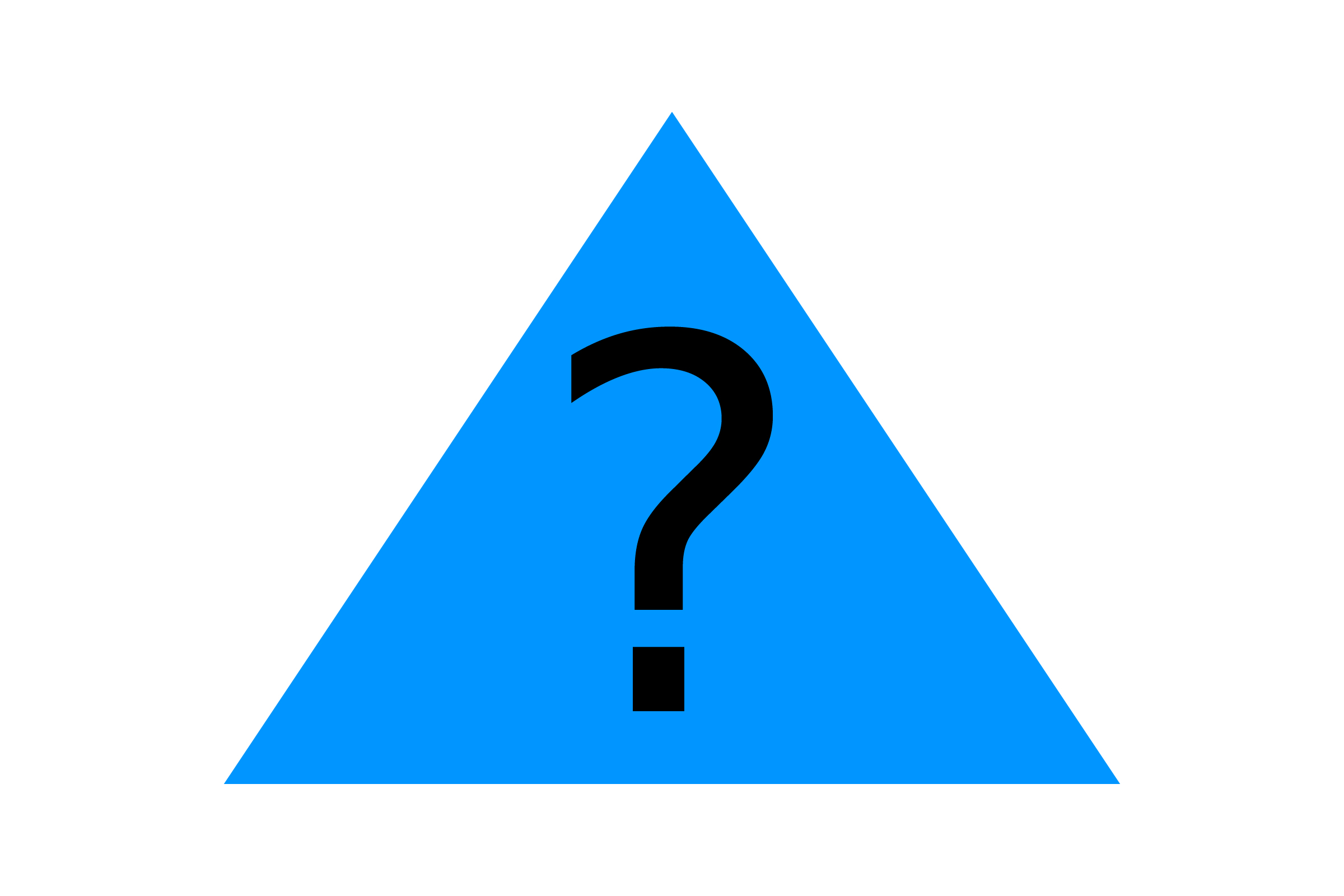# WHERE ARE THE OTHER TRIANGLE EXAMPLES?

You might be wondering where all the other triangle collision examples are. The answer: we've already made them! The smallest number of sides a polygon can have is three, so all the polygon code will work for triangles too.

However, since our polygon code is meant to work with PVectors, you will need to define your triangles using three PVector objects, or modify the collision functions.

NEXT: Section 5 Challenges